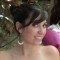Home » Java Basics » arrays » Java 2D Array Example

### About Katerina ZamaniKaterina has graduated from the Department of Informatics and Telecommunications in National and Kapodistrian University of Athens (NKUA) and she attends MSc courses in Advanced Information Systems at the same department. Currently, her main academic interests focus on web applications, mobile development, software engineering, databases and telecommunications.

# Java 2D Array Example

Java give us the opportunity of using arrays with many dimensions. The most common multi-dimensional arrays that are used in java apps, are one-, two- and three-dimensional.

In this example we are going to present some ways of creating 2D arrays, which are represented as tables with row and column style.

## 1. Example of 2d array

We are going to define 3 types of 2D arrays, where the first represents a table of `Integers`, the second one is an array of an array that includes objects and the third one constitutes a simple array with initial values.

Create a java class named `TwoDarrayClass` and paste the following code.

TwoDarrayClass.java:

```package com.javacodegeeks.javabasics.twoDarray;

public class TwoDarrayClass {

static final int ROWS = 4;
static final int COLS = 2;

private int num;
private String name;

public TwoDarrayClass(int num, String name) {
super();
this.num = num;
this.name = name;
}

public int getNum() {
return num;
}
public String getName() {
return name;
}

public static void main(String[] args) {

// first way of 2d array
int[][] tableInt = new int[ROWS][COLS];

// put values into the tableInt
for(int i=0; i<ROWS; i++) {
for(int j=0; j<COLS; j++) {
tableInt[i][j] = i+j;
}
}
// retrieve all values of table using foreach
String outputTable1="Values of tableInt:\n";
for (int[] row : tableInt) {
for (int val : row) {
outputTable1 += " " + val;
}
outputTable1 += "\n";
}
System.out.println(outputTable1);

// second way of 2d array, using a class
TwoDarrayClass[][] tableClass;
// array of references to arrays
tableClass = new TwoDarrayClass[ROWS][];

for(int i=0; i<ROWS; i++) {
tableClass[i] = new TwoDarrayClass[COLS];
}

// create the object into the tableClass
for(int i=0; i<tableClass.length; i++) {
for(int j=0; j<tableClass[i].length; j++) {
tableClass[i][j] = new TwoDarrayClass(i+j, "row"+i+"-col"+j);
}
}

// print the name of the class of the tableClass
String outputTable2 = "The values of tableClass:\n";
for (int row = 0; row < tableClass.length; row++) {
for (int col = 0; col < tableClass[row].length; col++) {
outputTable2 += " name = " + tableClass[row][col].getName()+ "|";
}
outputTable2 += "\n";
}
System.out.println(outputTable2);

// a more static way...
String[][] fruitsTable = new String[][] {
{"apple","orange"},
{"kiwi","strawberry"},
{"peach","melon"} ,
{"cranberry","grape"}
};
System.out.println("Fruits in winter are: "+fruitsTable+" and "+fruitsTable);
System.out.println("Fruits in summer are: "+fruitsTable+" and "+fruitsTable);

}

}
```

Now, lets explain the code above. First of all we define some name constants that represent the number of rows and columns of the 2D arrays. That makes our code more secure, flexible and easily reused. The first 2-dimensional array is a table of `int` (`tableInt`), with 4 rows and 2 columns. To insert values to the this matrix, we use nested loops which go through all the positions of the array. Nested loops can also be used in order to retrieve all data from the array. Another way of processing the array, and that we use in the example too, is the utilize of `foreach` loop.

Instead of declaring only primitive types in an array, we can use objects too. In our example this case is covered by the definition of `tableClass` 2D array. Every cell of this 2-dimensional array represents an instance of `TwoDarrayClass` class. In this situation, we create the reference of the 2D array first, in addition we create the arrays that reference to the arrays and finally, in the loop, we create the array of references to the object.

The third part of this tutorial represents a more static 2D array of Strings. As you can notice, this manner is very similar to the declaration of one-dimensional array. The third way allocates again a table with 4 rows and 2 columns.

For a better understanding, you can see the output of the execution of the above code.

Output:

``````Values of tableInt:
0 1
1 2
2 3
3 4

The values of tableClass:
name = row0-col0| name = row0-col1|
name = row1-col0| name = row1-col1|
name = row2-col0| name = row2-col1|
name = row3-col0| name = row3-col1|

Fruits in winter are: apple and orange
Fruits in summer are: peach and melon```
```

This was a tutorial about 2D array in java. Download thesource code of this example: 2DarrayExample.zip(No Ratings Yet)
Start the discussion Views Tweet it!

# Do you want to know how to develop your skillset to become a Java Rockstar?

## Subscribe to our newsletter to start Rocking right now!

### and many more ....